# 15 Excel Data Analysis Functions You Need to Know

If you’ve ever used Excel, then you’ve probably experienced the agony of choosing an incorrect formula to analyze a data set. Maybe you worked on it for hours, finally giving up because the data output was wrong or, the function was too complicated, and it seemed more straightforward to count the data yourself manually. If that sounds like you, then this Data Analysis in Excel top 15 is for you.

There are hundreds of functions in Excel, and it can be overwhelming trying to match the right formula with the right kind of data analysis. The most useful functions don’t have to be complicated. Fifteen simple functions will improve your ability to analyze data, making you wonder how you ever lived without them.

Whether you dabble in Excel or use it heavily at your job, there is a function for everyone in this list.

## 1. CONCATENATE

=CONCATENATE is one of the easiest to learn but most powerful formulas when conducting data analysis. Combine text, numbers, dates and more from multiple cells into one. That is an excellent function for creating API endpoints, product SKUs, and Java queries.

Formula:

#### =CONCATENATE(SELECT CELLS YOU WANT TO COMBINE)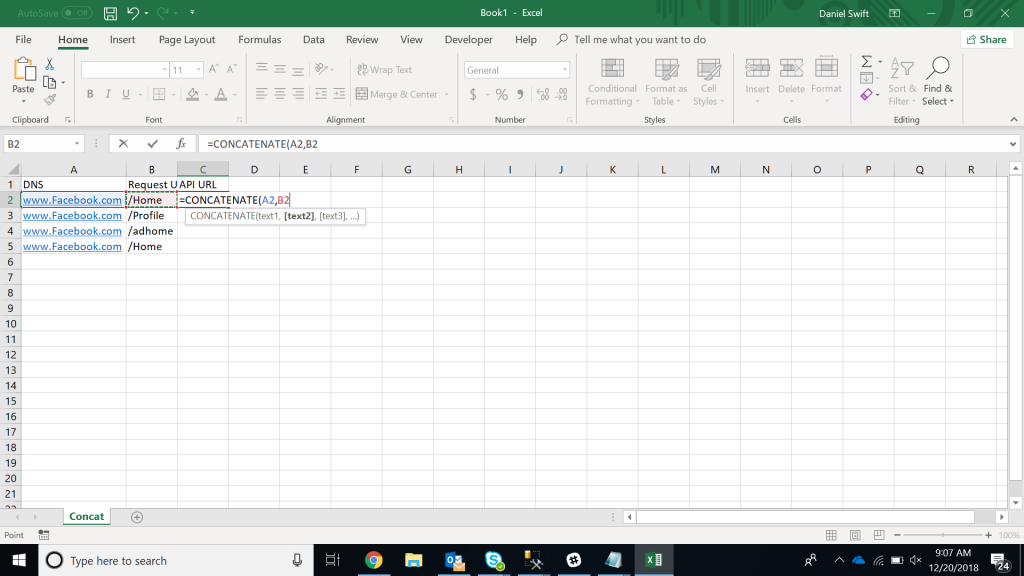In this example:

## 2. LEN

=LEN quickly provides the number of characters in a given cell. As in the example above, you can identify two different kinds of product Stock Keeping Units (SKUs) using the =LEN formula to see how many characters the cell contains. LEN is especially useful when trying to determine the differences between different Unique Identifiers (UIDs), which are often lengthy and not in the right order.

Formula:

#### =LEN(SELECT CELL)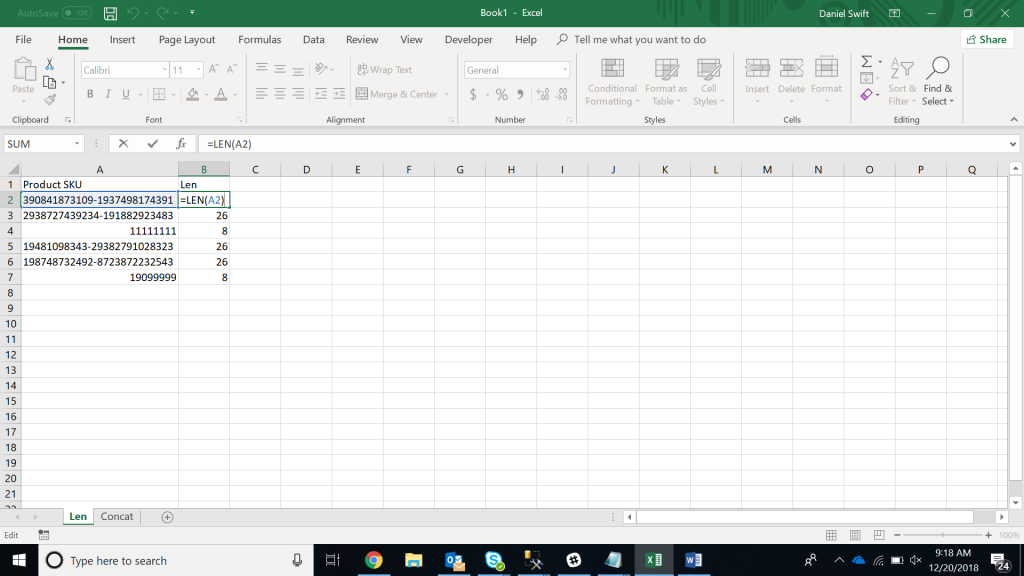In this example:

## 3. COUNTA

=COUNTA identifies whether a cell is empty or not. In the life of a data analyst, you’re going to run into incomplete data sets daily. COUNTA will allow you to evaluate any gaps the dataset might have without having to reorganize the data.

Formula:

#### =COUNTA(SELECT CELL)In the example:

## 4. DAYS/NETWORKDAYS

=DAYS is precisely what it implies it is. This function determines the number of calendar days between two dates. It is a useful tool for assessing the lifecycle of products, contracts, and run rating revenue depending on service length – a data analysis essential.

=NETWORKDAYS is slightly more robust and useful. This formula determines the number of “workdays” between two dates as well as an option to account for holidays. Even workaholics need a break now and then! Using these two formulas to compare time frames is especially helpful for project management.

Formulas:

OR

#### =NETWORKDAYS(SELECT CELL, SELECT CELL,[numberofholidays])

note: [numberofholidays] is optionalIn the example:

OR

## 5. SUMIFS

=SUMIFS is one of the “must-know” formulas for a data analyst. The standard method used is =SUM, but what if you need to sum values based on multiple criteria? SUMIFS is it. In the example below, SUMIFS is used to determine how much each product is contributing to top-line revenue.

Formula:

#### =SUMIF(RANGE,CRITERIA,[sum_range])

note: [sum_range] is optional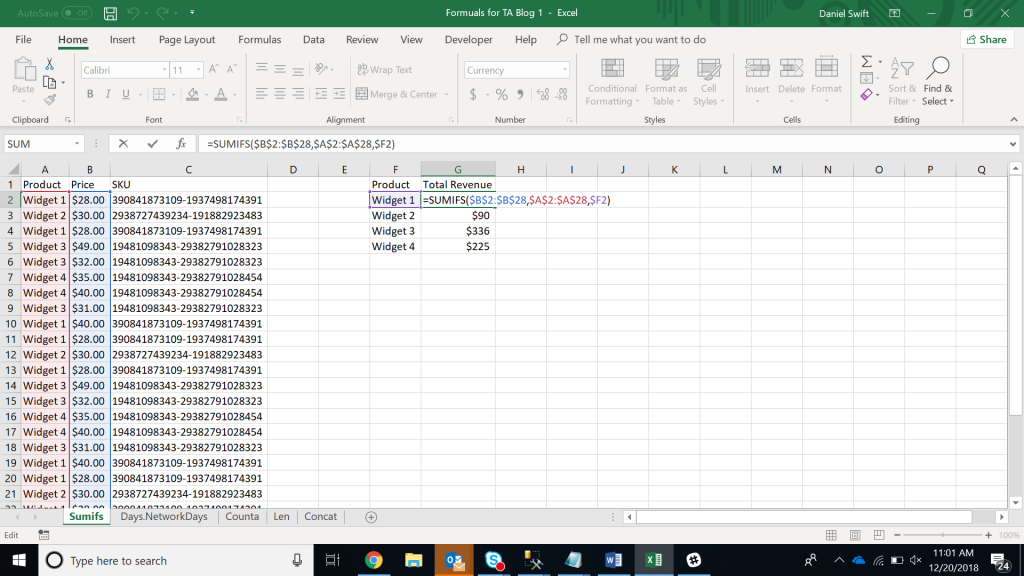In the example:

## 6. AVERAGEIFS

Much like SUMIFS, AVERAGEIFS allows you to take an average based on one or more criteria.

Formula:

#### =AVERAGEIF(SELECT CELL, CRITERIA,[AVERAGE_RANGE])

note: [average_range] is optionalIn the example:

## 7. VLOOKUP

VLOOKUP is one of the most useful and recognizable data analysis functions. As an Excel user, you’ll probably need to “marry” data together at some point. For example, accounts receivable might know how much each product costs, but the shipping department can only provide units shipped. That is the perfect use case for VLOOKUP.

In the image below, we use reference data (A2) combined with the pricing table to have excel looking up matching criteria in the first column and returning an adjacent value.

Formula:

#### =VLOOKUP(LOOKUP_VALUE,TABLE_ARRAY,COL_INDEX_NUM, [RANGE_LOOKUP])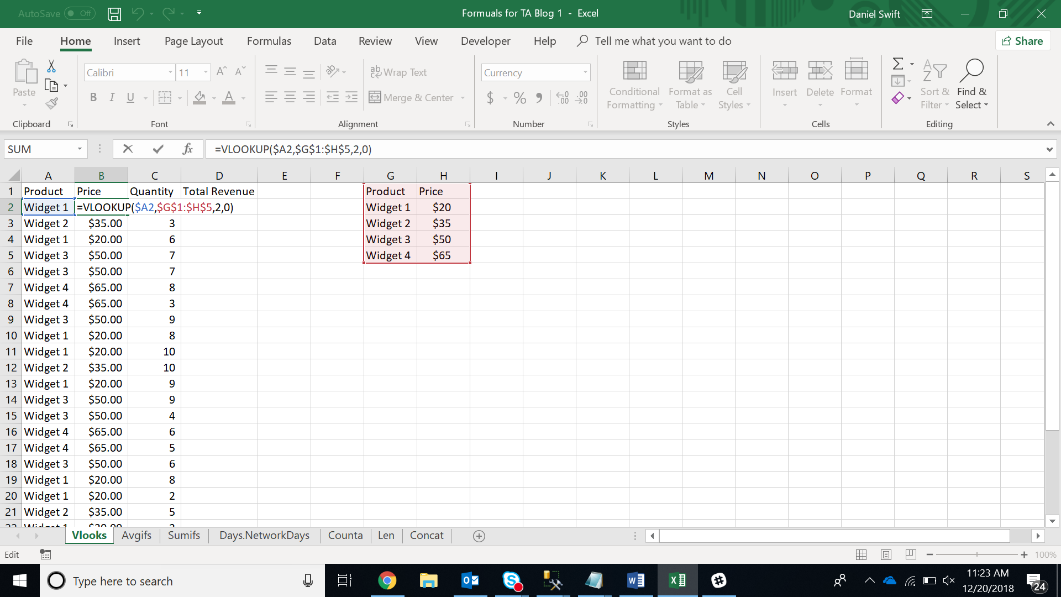In the example:

## 8. FIND/SEARCH

=FIND/=SEARCH are powerful functions for isolating specific text within a data set. Both are listed here because =FIND will return a case-sensitive match, i.e. if you use FIND to query for “Big” you will only return Big=true results. But a =SEARCH for “Big” will match with Big or big, making the query a bit broader. This is particularly useful for looking for anomalies or unique identifiers.

Formula:

#### =FIND(TEXT,WITHIN_TEXT,[START_NUMBER]) OR =SEARCH(TEXT,WITHIN_TEXT,[START_NUMBER])

note: [start_number] is optional and is used to indicate the starting cell in the text to search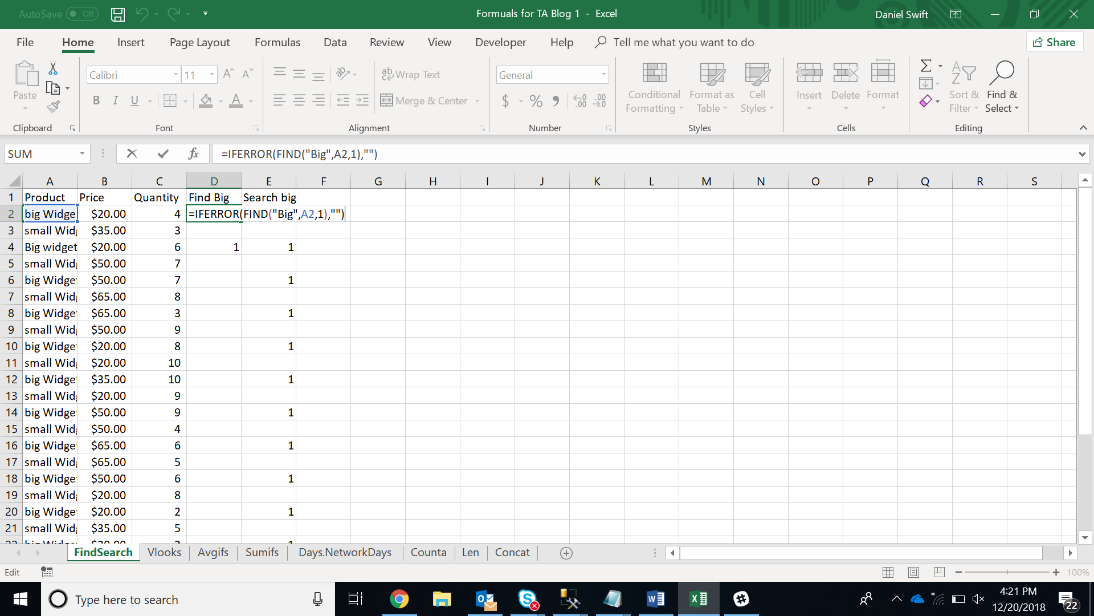In the example:

## 9. IFERROR

=IFERROR is something that any analyst who actively presents data should take advantage of. Using the previous example, looking for specific text/values in a dataset won’t return a match. That causes a #VALUE error, and while harmless, it is distracting and an eyesore.

Use =IFERROR to replace the #VALUE errors with any text/value. In the example above, the cell is blank so that data consumers can easily pick out which rows returned a matching value.
Formula:

#### =IFERROR(FIND“VALUE”,SELECT CELL,VALUE_IF_ERROR)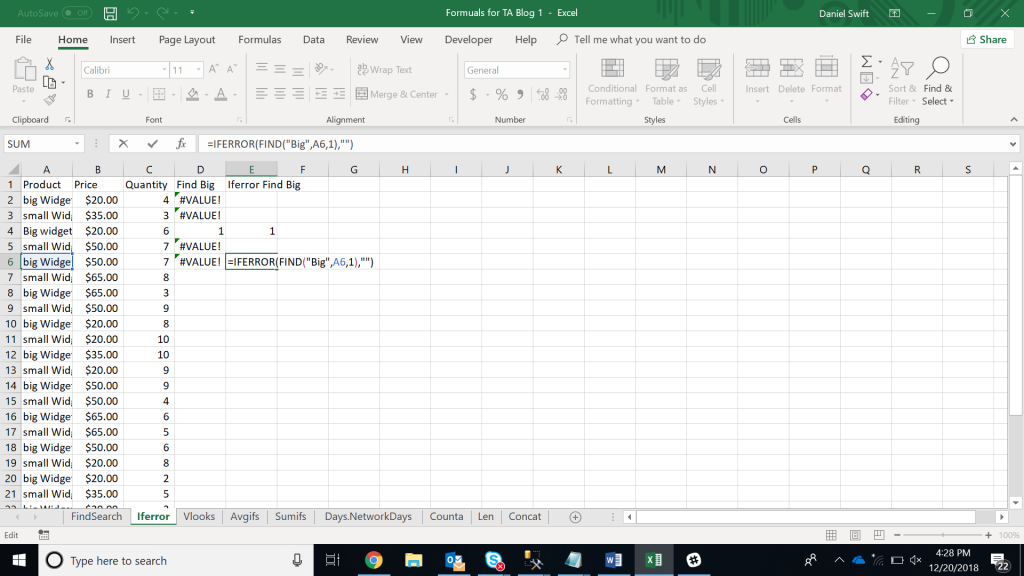In the example:

## 10. COUNTIFS

=COUNTIFS is the easiest way to count the number of instances a dataset meets a set of criteria. In the example above the product name is used to determine which product was the best seller. COUNTIFS is powerful because of the limitless criteria you can input.
Formula:

#### =COUNTIFS(RANGE,CRITERIA)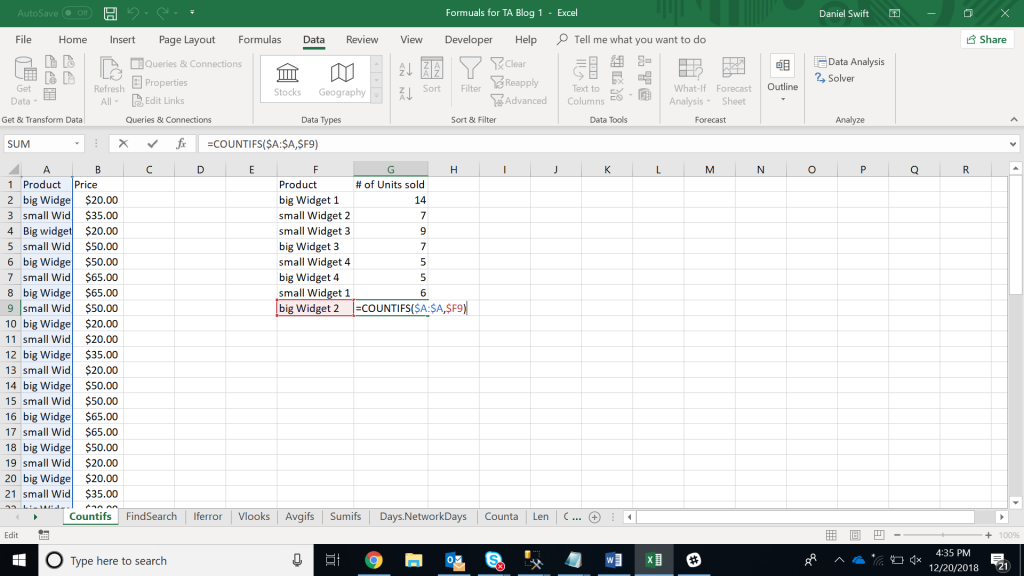In the example:

## 11. LEFT/RIGHT

=LEFT, =RIGHT are efficient and straightforward methods for extracting static data out of cells. =LEFT will return the “x” number of characters from the beginning of the cell, while =right will return the “x” number of characters from the end of the cell. In the example below, =LEFT is used to extract the consumer’s area code from their phone number, while =RIGHT is used to extract the last four digits.

Formula:

OR

#### =RIGHT(SELECT CELL,NUMBER)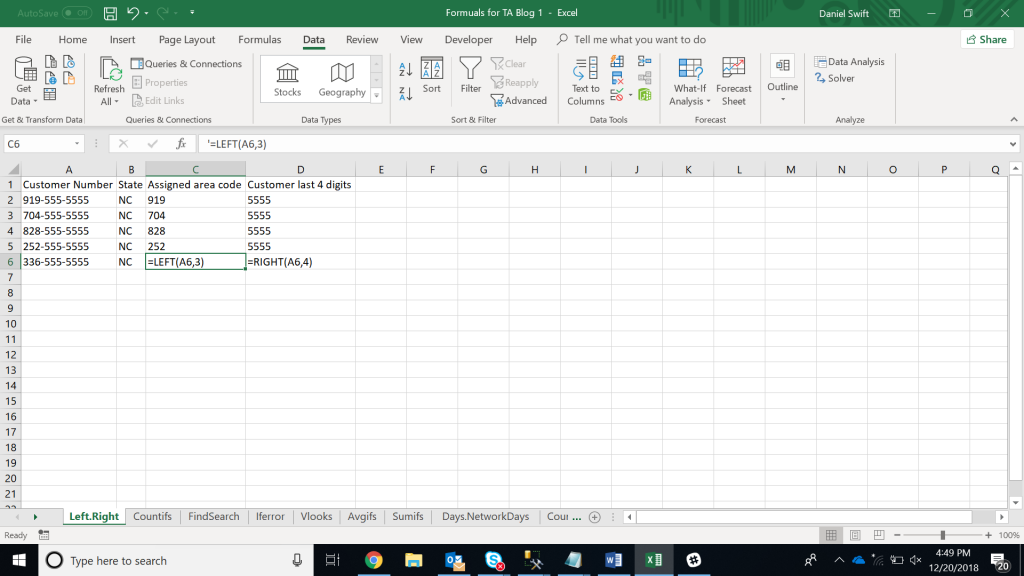In this example:

AND

## 12. RANK

=RANK is an ancient excel function, but that doesn’t downplay its effectiveness for data analysis. =RANK allows you to quickly denote how values rank in a dataset in ascending or descending order. In the example, RANK is being used to determine which clients order the most product.

Formula:

#### =RANK(SELECT CELL,RANGE_TO_RANK_AGAINST,[ORDER])

note: [order] is optional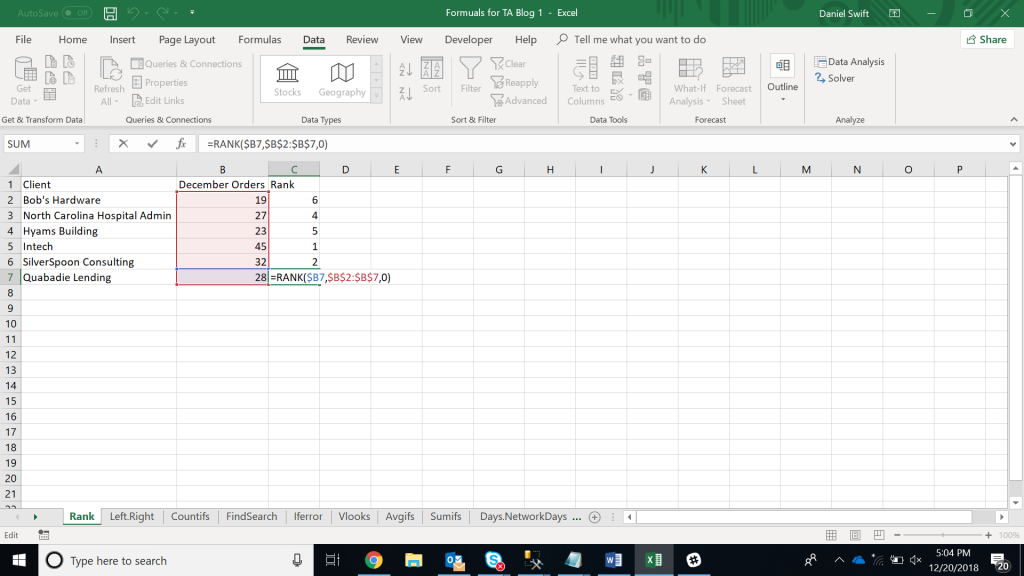In the example:

#### =RANK(\$B7,\$B\$2:\$B\$7,0)

note: 0 returns the largest value ranked #1

## 13. MINIFS

=MINIFS is very similar to the min function except it allows you to take the minimum of a set of values, and match on criteria as well. In the example, =MINIFS is used to find the lowest price each product sold for.

Formula:

#### =MINIFS(RANGE1,CRITERIA1,RANGE2)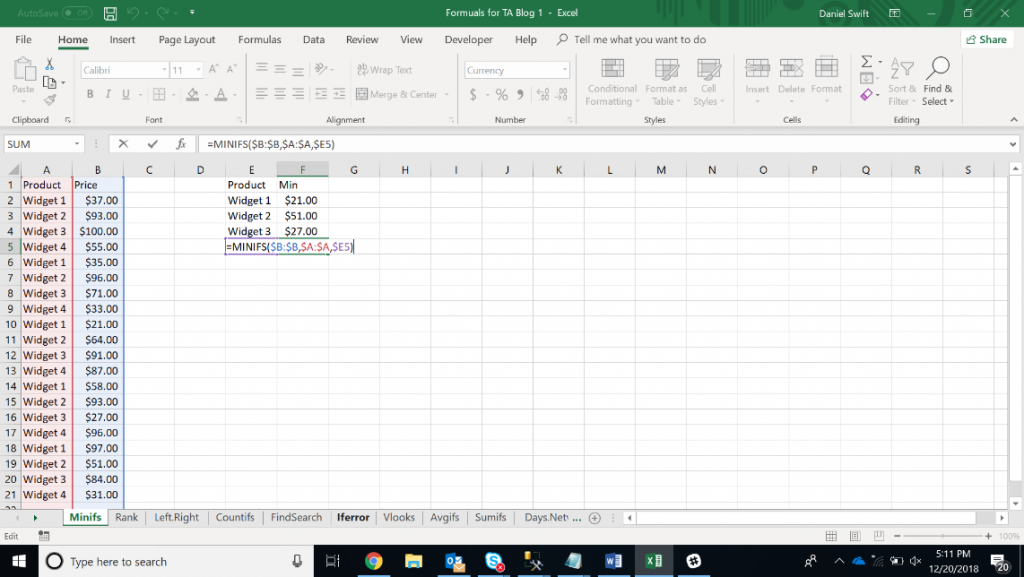In this example:

## 14. MAXIFS

=MAXIFS, like its counterpart minifs, allows you to match on criteria, but this time it looks for the maximum number.
Formula:

#### =MAXIFS(RANGE1,CRITERIA1,RANGE2)In this example:

## 15. SUMPRODUCT

=SUMPRODUCT is an excellent function to calculate average returns, price points, and margins. SUMPRODUCT multiples one range of values by its corresponding row counterparts. It’s data analysis gold. In the example below, we calculate the average selling price of all our products by using sumproduct to times Price by Quantity and then divide by the total volume sold.

Formula:

#### =SUMPRODUCT(RANGE1,RANGE2)/SELECT CELL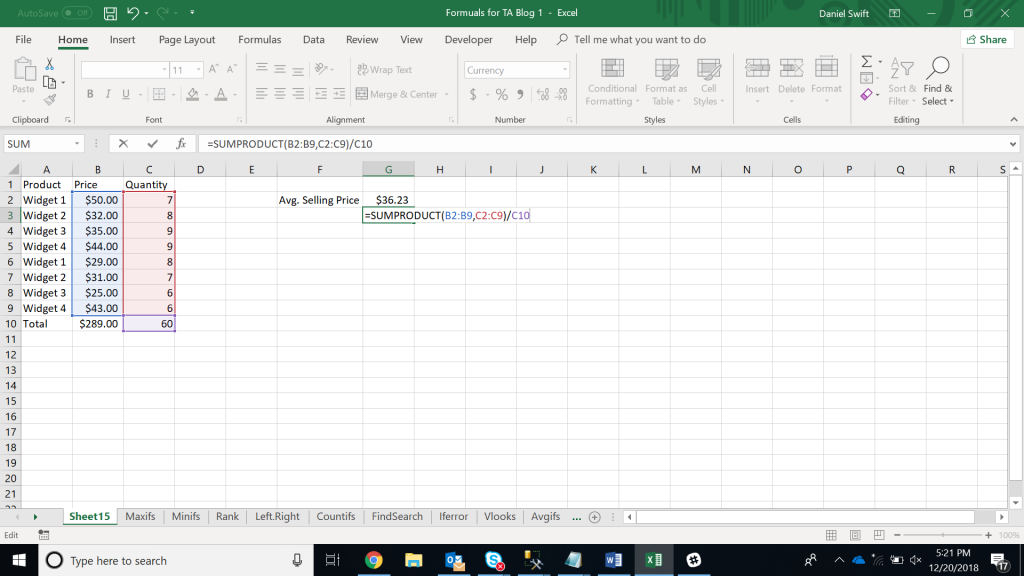In this example:

#### =SUMPRODUCT(B2:B9,C2:C9)/C10

We hope you found that useful. If you’re interested in Data Analysis in Excel, take a look at the Excel course that has helped hundreds of thousands of people master Excel.Data Analysis in Excel
£49.00Microsoft Excel
£49.00Ultimate Microsoft Excel Bootcamp Bundle
£156.80